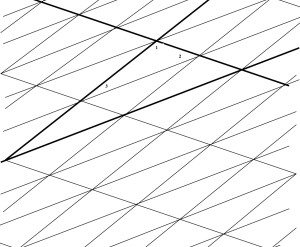This diagram shows 3 sets of parallel lines. What do you notice in the diagram?

Look at the triangle with angles marked 1, 2 and 3.

Call this T.

Label angles equal to these angles. Why are they equal?

Look at the larger heavily outlined triangle.

How many copies of triangle T can you see in this triangle?